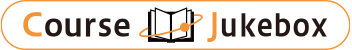### CourseDetailDegree
Bachelor
Course delivery methods
face-to-face
Subject
Mathematical sciences
Program
School
College of Science
Department
Campus
Main Campus
Classroom
Course Offering Year
Course Offering Month
February - June
Weekday and Period
Wednesday 8,9,10 Friday 1,2
Capacity
100
Credits
4
Language
English
Course Number
MATH1202 (201E101A2)

### Calculus (general Mathematics) (a)(2) National Taiwan University

#### Course Overview

We first summarize what we have learned in the last semester to find the Taylor expansion of a given function. This has tremendous applications in all kinds of engineering. The single variable calculus ends here. Then we move on to calculus in severable variables. The approach is similar to what we have done in the last semester: limit, derivative, optimization problem by using derivatives (Lagrange multipliers), integrals, then to ''Fundamental Theorem of Calculus.'' The formulas of FTC in two and three variables in the format of Green-Stokes and Divergence Theorems is technical to explain and learn. However, it all says that the integral of a function in the interior is exactly the total change on the boundary, when interpreted in a suitable sense.

#### Learning Achievement

1. Taylor expansion 2. Calculus in two and three variables: limit and derivative 3. Optimization problem: Lagrange multipliers 4. Integrals in two and three variables 5. Green-Stokes and divergence theorems.

#### Course prerequisites

Single variable calculus: limit, derivative, integral. Idea of linear approximation.

Lai, Ching-Jui

#### Other information

*Majors-only (including minor and double major students).
(College of Science) Department of Chemistry,
(College of Science) Department of Atmospheric Sciences,
(College of Science) Department of Geography,
(College of Engineering) Department of Mechanical Engineering,
(College of Engineering) Department of Chemical Engineering